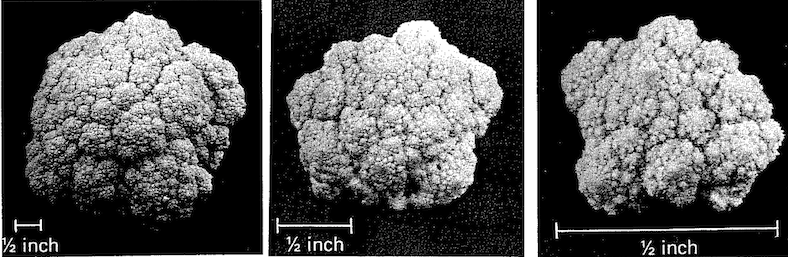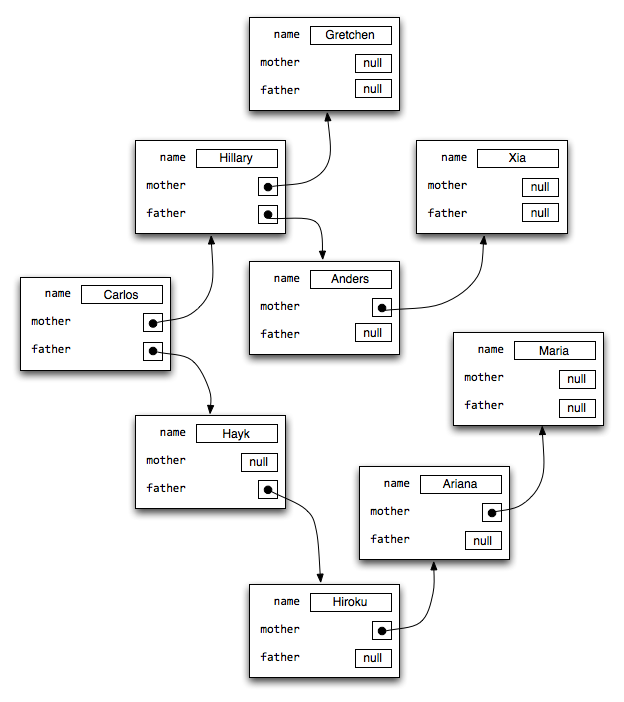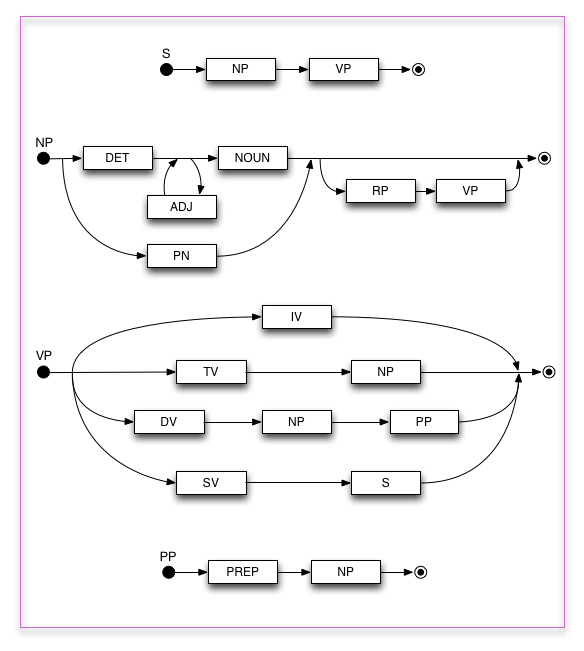# Recursion

Recursion is important.

## What is Recursion?

Recursion is the process of repeating in a self-similar fashion. Objects that contain self-similar smaller "copies" of themselves are recursive.

## Why is it Important?

Recursion is the way that the infinite can arise from a finite description. Or more usefully, it is the way that nature can use extremely compact representations for seemingly unbounded, complex phenomena.

Just look around. You see it everywhere:

• In nature
• In math
• In computer science
• In language
• In art
• In music

## Recursion in Nature

Self-similarity occurs everywhere in nature, it seems. Zoom in on coastlines, clouds, broccoli, plants, fire (at least for a few levels) and things look the same.Objects that are self-similar to many (even an infinite number of) levels are called Fractals and can be generated by repeated application of rather simple rules. See:

## Recursion in Math

Finite sets can be defined by enumerating their elements, but infinite sets cannot. We can build up infinite sets via rules.

### Recursive Definitions

Natural Number
• 0 is a natural number
• If n is a natural number then so is n+1
• Nothing else is a natural number
Odd integer
• 1 is an odd integer
• If k is a an odd integer then so are k+2 and k-2
• Nothing else is an odd integer
Palindrome
• A string of length 0 or 1 is a palindrome
• If p is a palindrome and c is a character then cpc is a palindrome
• Nothing else is a palindrome
Polynomial
• A real number is a polynomial
• The variable x is a polynomial
• If p and q are polynomials then so are p+q, p-q, pq, p/q, and (p).
• Nothing else is a polynomial
Propositional Formula (PF)
• p, q, r, s, p', q', r', s', p'', ... are PFs
• If A and B are PFs then so are ~A, A ∧ B, A ∨ B, and (A).
• Nothing else is a PF
Ancestors of x
• Mother(x) is in Ancestors(x)
• Father(x) is in Ancestors(x)
• if y is in Ancestors(x) then so are Mother(y) and Father(y)
• Nothing else is in Ancestors(x)

Note the importance of the "nothing else" clauses; without them your definitions are not unique.

Exercise: Give recursive definitions for the descendants of a person, and for the notion of a list of objects.

### Recursive Definitions vs. Circular Definitions

Proper recursive definitions must have a basis — a part of the definition that does not rely on the recursive "step." Without a basis, you might get into serious trouble, and may even end up with a true circular definition.

x =def cos(x)
You got lucky here; this uniquely defines x
x =def x
ERROR: This is a circular definition, and therefore does not define anything!
x =def x + 1
ERROR: There's no such x, assuming we're taking about finite numbers here
x =def 6 - x2
DUBIOUS: x could be 2 or -3

### Generating Sequences with Recursion

Infinite sequences are described finitely by giving a rule (or rules) for generating the next value in the sequence from previous ones. Examples:

• Fibonacci Numbers: F(0) = 1, F(1) = 1, F(n+2) = F(n) + F(n+1)
• Lucas Numbers: L(0) = 2, L(1) = 1, L(n+2) = L(n) + L(n+1)
• Catalan Numbers: C(0) = 1, C(n+1) = sum for i=0 to n of C(i)*C(n-i)
• ... and many more

### Inductive Proofs

Suppose you wanted to prove that every element in a recursively defined set had some property. You can't prove the property for each element because there are an infinite number of elements. But you can prove the property for the basis elements and then show that elements generated by the recursive rules maintain the property whenever the elements from which they were generated have the property. Example:

Prove: The successor of every odd number is divisible by 2.
Proof: Basis: The successor of 1 is 2 and 2 is divisible by 2. Step: Assume k is odd and k+1 is divisible by 2. We have to show under these assumptions that (1) the successor of k-2 is divisible by 2 and (2) the successor of k+2 is divisible by 2. To prove (1) we note that the successor of k-2 is k-1 which is divisible by 2 because k-1 = k+1-2. To prove (2) note that the successor of k+2 is k+3 which is divisible by 2 because k+3 = k+1+2. This completes the proof.

## Recursion in Computer Science

We see recursion in both algorithms and in data.

### Recursive Algorithms

Here are three examples, out of the zillions known:

To walk n steps:

1. If n <= 0, stop
2. Take a single step
3. Walk n-1 steps

To walk to the wall:

1. If you are at the wall, stop
2. Step
3. Walk to the wall

To sort a list:

1. If the list has zero or one elements, stop
2. Sort the first half
3. Sort the second half
4. Merge the two sorted halves together

### Recursive Functions

To write a recursive function, you must:

1. have a base case
2. ensure that each recursive call makes progress toward the base case

Here are some examples in Python:

recursionexamples.py
```# Here are a few examples of recursion.  The example functions were chosen
# because they are short and concise examples, not because they are good
# code.  In fact, nearly every method shown here is inferior to its
# iterative counterpart.

def factorial(n):
return 1 if n == 0 else n * factorial(n - 1)

def sum_of_digits(n):
if n < 0:
return sum_of_digits(-n)
elif n < 10:
return n
else:
return sum_of_digits(n / 10) + (n % 10);

def is_palindrome(s):
return len(s) <= 1 or (s == s[-1] and is_palindrome(s[1:-1]))

def gcd(x, y):
return x if y == 0 else gcd(y, x % y)
```

### Understanding Recursive Functions

It is strongly suggested that you evaulate recursive functions by hand to get comfortable with them. For example:

```is_palindrome("racecar")
= ('r' == 'r') and is_palindrome("aceca")
= true and is_palindrome("aceca")
= is_palindrome("aceca")
= ('a' == 'a') and is_palindrome("cec")
= true and is_palindrome("cec")
= is_palindrome("cec")
= ('c' == 'c') and is_palindrome("a")
= true and is_palindrome("a")
= is_palindrome("a")
= true
```
```factorial(4)
= 4 * factorial(3)
= 4 * (3 * factorial(2))
= 4 * (3 * (2 * factorial(1)))
= 4 * (3 * (2 * (1 * factorial(0))))
= 4 * (3 * (2 * (1 * 1)))
= 4 * (3 * (2 * 1))
= 4 * (3 * 2)
= 4 * 6
= 24
```
```sumOfDigits(-48729)
= sumOfDigits(48279)
= sumOfDigits(4827) + 9
= (sumOfDigits(482) + 7) + 9
= ((sumOfDigits(48) + 2) + 7) + 9
= (((sumOfDigits(4) + 8) + 2) + 7) + 9
= (((4 + 8) + 2) + 7) + 9
= ((12 + 2) + 7) + 9
= (14 + 7) + 9
= 21 + 9
= 30
```
```gcd(444,93)
= gcd(93,72)
= gcd(72,21)
= gcd(21,9)
= gcd(9,3)
= gcd(3,0)
= 3
```

### Efficiency

Note that just because some functions are described recursively doesn't mean programmers should write them as such. Most programming languages let you describe recursive processes with loops, which run much faster. For example:

```def factorial(n):
result = 1
for i in range(n):
result *= i
return result
```

Often needless recursion turns linear into exponential complexity!

```# HORRIFIC CODE for computing the nth Fibonacci Number. NEVER write code like this.
def fib(n):
return 1 if n <= 1 ? else fib(n-2) + fib(n-1)
```

You can see why this inefficient:

```fib(4)
= fib(3) + fib(2)
= (fib(2) + fib(1)) + fib(2)
= ((fib(1) + fib(0)) + fib(1)) + fib(2)
= ((1 + fib(0)) + fib(1)) + fib(2)
= ((1 + 0) + fib(1)) + fib(2)
= (1 + fib(1)) + fib(2)
= (1 + 1) + fib(2)
= 2 + fib(2)
= 2 + (fib(1) + fib(0))
= 2 + (1 + fib(0))
= 2 + (1 + 0)
= 2 + 1
= 3
```

BTW it wil make 25 calls to fib just to determine that the value of fib(6) is 13, and 41 to get the value of fib(7).

Exercise: Trace out the evaluation of fib(6).
Exercise: How many calls are made when calling fib(n)? Answer as a function of n.

Here is another disastrous example:

```# Horrific Code for determining the minimum number of coins
# needed to make n cents where the coin values are stored in the
# array denominations.  The denominations array must contain
# a 1 in it, or the result of this method is undefined.
def minimum_coins(n, denominations)
int min_so_far = n
for d in denominations:
if d == n:
return 1
elif d < n:
min_so_far = min(min_so_far, minimum_coins(n-d, denominations))
return min_so_far
```
Exercise: Just how many calls to minimumNumberOfCoins are made when calling the method with the arguments 97 and [4, 1, 6, 23, 11]?

### Recursive Datatypes

Often you'll have a datatype whose components are (references to) objects of the datatype itself. For example, a representation of a person can have a name, a birthdate, a mother, and a father. The mother and father are ... people!Most of the methods operating on such an object would naturally be recursive:

```function length_of_known_maternal_line(p) {
return p === null ? 0 : 1 + length_of_known_maternal_line(p.mother);
}
```

## Recursion in Language

Languages can exhibit recursion. For example, here is a grammar for a subset of English (the "|" means "or," the "*" means "zero-or-more," and the "?" means "zero-or-one."):

```S      →  NP VP
NP     →  (PN | DET ADJ* NOUN) (RP VP)?
VP     →  IV | TV NP | DV NP PP | SV S
PP     →  PREP NP
PN     →  grace | donald | alan | she
DET    →  a | the | his | her
NOUN   →  doctor | dog | rat | girl | toy
RP     →  who | that
ADJ    →  blue | heavy | fast | new
PREP   →  to | above | around | through
IV     →  fell | jumped | swam
TV     →  liked | knew | hit | missed
DV     →  gave | threw | handed
SV     →  dreamed | believed | thought | knew
```

In syntax diagrams, also known as recursive transition networks (RTNs):Here sentences contain verb phrases which contain sentences. Noun phrases contain verb phrases which contain verb phrases. Because of recursion we can make sentences of arbitrary length. Here's a simple example:

```S
NP VP
DET NOUN RP VP VP
the NOUN RP VP VP
the dog RP VP VP
the dog that VP VP
the dog that SV S VP
the dog that thought S VP
the dog that thought NP VP VP
the dog that thought PN VP VP
the dog that thought grace VP VP
the dog that thought grace TV NP VP
the dog that thought grace hit NP VP
the dog that thought grace hit PN VP
the dog that thought grace hit alan VP
the dog that thought grace hit alan DV NP PP
the dog that thought grace hit alan threw NP PP
.
.
.
the dog that thought grace hit alan threw the new blue toy to the fast rat
```
Exercise: Draw the parse tree for this derivation.

Here's a construction that makes clear that you really can go on as long as you like:

```S
NP VP
NP SV S
NP SV NP VP
NP SV NP SV S
NP SV NP SV NP VP
NP SV NP SV NP SV S
.
.
.
she dreamed she dreamed she dreamed she dreamed . . . she dreamed the dog swam
```

## Recursion in Art

Sometimes you'll see a drawing contain a copy of itself. This is called the Droste Effect.

This effect appears in Escher's Print Gallery, though Escher did not draw the recursive effect himself. See how it was added.